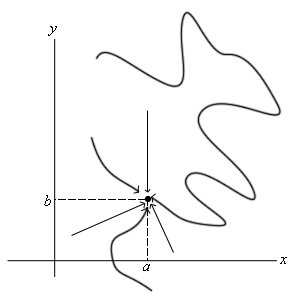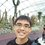# Multivariable limitsRecently, I've just learn about multivariate limits in my Advanced Calculus class. The concept is such that there are infinite possible approaches in solving limit problems (see picture). Why would it not be sufficient to just consider the direct displacement of our approach? If there is a direct displacement from all directions, it would form an area such that all paths taken falls within the area. Hence, if the limit of the direct displacement exists, we can safely conclude that the limits of all paths exists.

If that is the case, we can simply let $y=mx, m\in\mathbb{R}$ and solve the limits. I will show my approach in multivariate problems in the following forms. Consider $4$ cases, $\lim_{(x, y)\rightarrow(0, 0)}f(x, y)$, $\lim_{(x, y)\rightarrow(a, 0)}f(x, y)$, $\lim_{(x, y)\rightarrow(0, b)}f(x, y)$ and $\lim_{(x, y)\rightarrow(a, b)}f(x, y)$ where $a, b\neq0$. In all cases, let $y=mx$.

Case $1$: $\lim_{(x, y)\rightarrow(0, 0)}f(x, y)$ $\because x, y\rightarrow0, \therefore m=\mathbb{R}$ $\therefore\lim_{(x, y)\rightarrow(0, 0)}f(x, y)=\lim_{(x, mx)\rightarrow(0, 0)}f(x, mx)\Rightarrow \lim_{(x, y)\rightarrow(0, 0)}f(x, y)=\lim_{x\rightarrow0}f(x, mx)$

If the multivariate limit exists, then $\lim_{(x, y)\rightarrow(0, 0)}f(x, y)=\lim_{x\rightarrow0)}f(x, mx)=L, L\in\mathbb{R}$, Else, $\lim_{x\rightarrow0}f(x, mx)=g(m)$ and the limit does not exist.

Case $2$: $\lim_{(x, y)\rightarrow(a, 0)}f(x, y)$ $\because x\rightarrow a, y\rightarrow0, \therefore m\rightarrow0$ $\therefore\lim_{(x, y)\rightarrow(a, 0)}f(x, y)=\lim_{(x, m)\rightarrow(0, 0)}f(x, mx)\Rightarrow\text{Case 1}$ And repeat the process of case $1$ to obtain the answer.

Case $3$: $\lim_{(x, y)\rightarrow(0, b)}f(x, y)$ $\because x\rightarrow0, y\rightarrow b, \therefore m\rightarrow\frac{b}{0}\rightarrow\infty$ $\therefore\lim_{(x, y)\rightarrow(0, b)}f(x, y)=\lim_{(x, m)\rightarrow(0, \infty)}f(x, mx)$ Then, let $m=\frac{1}{t}$. Hence, $t\rightarrow0$ as $m\rightarrow\infty$. This gives us $\lim_{(x, m)\rightarrow(0, \infty)}f(x, mx)=\lim_{(x, \frac{1}{t})\rightarrow(0, 0)}f(x, \frac{x}{t})\Rightarrow\text{Case 1}$

Case $4$: $\lim_{(x, y)\rightarrow(a, b)}f(x, y)$ $\because x\rightarrow a, y\rightarrow b, \therefore m=\frac{b}{a}$ $\therefore\lim_{(x, y)\rightarrow(a, b)}f(x, y)=\lim_{x\rightarrow a}f(x, \frac{b}{a}x)$

Note: Reducing the multivariate limits to a single variable limit allows the use of L'hopital's Rule. I have tested on many (but not all) problems to prove that the method works.Note by Shaun Loong
6 years, 11 months ago

This discussion board is a place to discuss our Daily Challenges and the math and science related to those challenges. Explanations are more than just a solution — they should explain the steps and thinking strategies that you used to obtain the solution. Comments should further the discussion of math and science.

When posting on Brilliant:

• Use the emojis to react to an explanation, whether you're congratulating a job well done , or just really confused .
• Ask specific questions about the challenge or the steps in somebody's explanation. Well-posed questions can add a lot to the discussion, but posting "I don't understand!" doesn't help anyone.
• Try to contribute something new to the discussion, whether it is an extension, generalization or other idea related to the challenge.

MarkdownAppears as
*italics* or _italics_ italics
**bold** or __bold__ bold
- bulleted- list
• bulleted
• list
1. numbered2. list
1. numbered
2. list
Note: you must add a full line of space before and after lists for them to show up correctly
paragraph 1paragraph 2

paragraph 1

paragraph 2

[example link](https://brilliant.org)example link
> This is a quote
This is a quote
    # I indented these lines
# 4 spaces, and now they show
# up as a code block.

print "hello world"
# I indented these lines
# 4 spaces, and now they show
# up as a code block.

print "hello world"
MathAppears as
Remember to wrap math in $$ ... $$ or $ ... $ to ensure proper formatting.
2 \times 3 $2 \times 3$
2^{34} $2^{34}$
a_{i-1} $a_{i-1}$
\frac{2}{3} $\frac{2}{3}$
\sqrt{2} $\sqrt{2}$
\sum_{i=1}^3 $\sum_{i=1}^3$
\sin \theta $\sin \theta$
\boxed{123} $\boxed{123}$